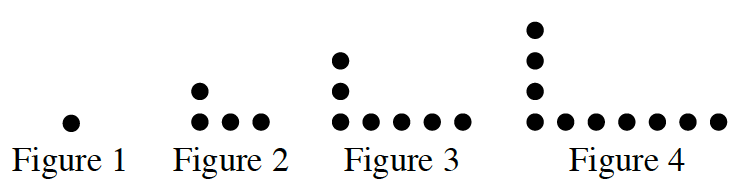### Home > CC1 > Chapter 6 > Lesson 6.1.4 > Problem6-55

6-55.

Copy the pattern below. Homework Help ✎1. Draw the fifth and sixth figures on your paper.

For each figure, $1$ vertical dot is added and $2$ horizontal dots are added.

2. Describe Figure 20.

How would you describe Figure 1? Figure 2?
Find a pattern so that you can imagine how Figure 20
will look, and then describe it in the same way you would Figures 1 and 2.

$20$ vertical dots and $39$ horizontal dots

3. How many dots will be in Figure 20?

The total number of dots in Figure 20 will be the sum of the vertical dots and the horizontal dots minus one (so that you do not count the corner dot twice).